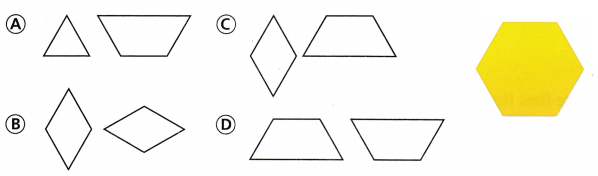Refer to our Texas Go Math Grade 3 Answer Key Pdf to score good marks in the exams. Test yourself by practicing the problems from Texas Go Math Grade 3 Module 15 Assessment Answer Key.

Vocabulary

Choose the best term from the box to complete the sentence.

Vocabulary
congruent
parallel lines
right angle
vertex

Question 1.
A __________ figures have the same size and shape. (p. 499)
Explanation:
Two shapes that are the same size and the same shape are congruent. They are identical in size and shape.

Question 2.
A __________ forms a square corner. (p. 481)
Explanation:
When two lines intersect to form a square corner. The intersection of two perpendicular lines forms a right angle or a 90° angle.

Question 3.
____________ appear to never cross or meet and are always the same distance apart. (p. 481)
Explanation:
Two or more lines that lie in the same plane and never intersect or meet each other are known as parallel lines.

Concepts and Skills

Circle all the words that describe the quadrilateral. (TEKS 3.6.A, 3.6.B)

Question 4.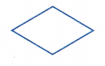rectangle
rhombus
parallelogram
square
Explanation:
Rhombus is a quadrilateral whose four sides all have the same length.

Question 5.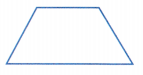parallelogram
rhombus
trapezoid
rectangle
Explanation:
A trapezoid is a quadrilateral with at least one pair of parallel sides.

Question 6.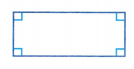rhombus
rectangle
square
parallelogram
Explanation:
A plane figure with four straight sides and four right angles, especially one with unequal adjacent sides, in contrast to a square.

Look at the first figure. Tell if it appears to be congruent to the second figure. Write yes or no. (TEKS 3.6.E)

Question 7.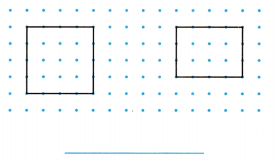Explanation:
The first figure is a square and the second figure is a rectangle
Two shapes that are the same size and the same shape are congruent. They are identical in size and shape

Question 8.Explanation:
The first figure and the second figure is same
Two shapes that are the same size and the same shape are congruent. They are identical in size and shape

Fill in the bubble for the correct answer choice.

Question 9.
What is one way you can sort the figures at the right? (TEKS 3.6.A)(A) 4 sides that are equal in length
(B) 2 pairs of opposite sides that are parallel
(C) 4 right angles
(D) exactly 1 pair of opposite sides that are parallel
Explanation:
2 pairs of opposite sides that are parallel is one way you can sort the figures at the right

Question 10.
Jennifer drew the quadrilaterals below. Which figure is NOT a rhombus? (TEKS 3.6.A, 3.6.B)Explanation:
Rhombus is a quadrilateral whose four sides all have the same length.
option C is not a rhombus

Question 11.
Which solid figure does NOT have a curved surface? (TEKS 3.6.A)